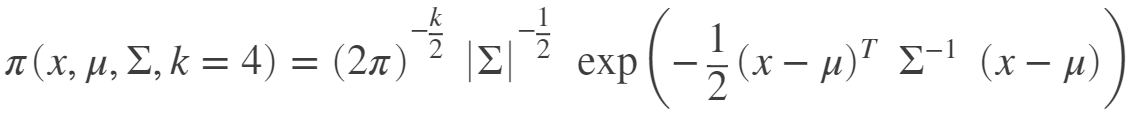Copyright (C) 2012-present, The Computational Data Science Lab
https://github.com/cdslaborg/paramonte
References
NOTE
If you are viewing an HTML version of this MATLAB live script on the web, you can download the corresponding MATLAB live script *.mlx file to this HTML page at,
https://github.com/cdslaborg/paramontex/blob/main/MATLAB/mlx/working_with_logarithm_of_objective_function/working_with_logarithm_of_objective_function.mlx?raw=true
This code, along with other MATLAB live scripts, is located at,
https://github.com/cdslaborg/paramontex/tree/main/MATLAB/mlx
Once you download the file, open it in MATLAB to view and interact with its contents, which is the same as what you see on this page.

# Why do we need to work with the logarithm of the mathematical objective functions?

In optimization and Monte Carlo sampling problems, since the mathematical objective functions (e.g., probability density functions) can take extremely small or large values, we often work with their natural logarithms instead. This is the reason behind the naming convention used in the ParaMonte library for the user's objective functions: getLogFunc, implying that the user must provide a function that returns the natural logarithm of the target objective function.
Why do we often prefer to work with the logarithm of functions in optimization and Monte Carlo sampling problems?
To find out, consider the following simple mathematical objective function getLogFunc_bad() that implements a 4-dimensional Standard MultiVariate Normal (MVN) distribution, whose generic Probability Density Function is given by the following equation (see also this Wikipedia article),

###NDIM = 4; % the number of dimensions of the domain of the objective function
getLogFunc_bad = @(point) log( mvnpdf( point ... The point at which the PDF of the multivariate normal distribution is computed. THIS IS A COLUMN VECTOR.
, [-10.; 15.; 20.; 0.0] ... This is the mean of the multivariate normal distribution. THIS IS A COLUMN VECTOR.
, [ 1.0, .45, -.3, 0.0 ...
; .45, 1.0, 0.3, -.2 ...
; -.3, 0.3, 1.0, 0.6 ...
; 0.0, -.2, 0.6, 1.0 ...
] ... This is the covariance of the multivariate normal distribution.
) ...
); % Note that the return value of getLogFunc must be always in natural logarithm.
Suppose we want to sample this MVN Probability Density Function (PDF) via the ParaMonte Monte Carlo simulation library. To do so, the ParaMonte library sampler routines will make repeated calls to this objective function that we have implmented in the above.
However, notice in the above implementation that we have suffixed the objective function with _bad. There are several good important reasons for such naming:
• The evaluation of this function involves a log(exp()) term in its definition. If the origin of the exp() term is not clear to you, take a look at the definition of the MVN distribution in the equation provided in the above. This is computationally very expensive and in general, is considered a bad implementation.
• The evaluation of the function as implemented in the above requires an inverse-covariance matrix computation on each call made to getLogFunc_bad(). This is completely redundant as the value of the covariance matrix -- and therefore, its inverse -- does not change throughout the simulation. Consequently, a lot of computational resources are wasted for nothing.
• The above implementation of the MVN distribution is quite prone to numerical overflow and underflow, which could cause the simulations to crash at runtime. For example, when the input value for point happens to be too far from the mean of the MVN distribution, it is likely for the resulting MVN PDF value to be so small that the computer rounds it to zero. Then log(0.0) in getLogFunc_bad() becomes undefined and the simulation crashes. That would only be the best-case scenario in which the crash will alert you about the problem. But more frequently, your code may not even complain that it is working with infinities and not doing any useful work.
It is, therefore, important to implement a numerically efficient, fault-tolerant MVN PDF calculator that resolves all of the above concerns. This is possible if we take a second look at the equation for the MVN PDF in the above and try directly implement its logarithm as our computational objective function,
NDIM = 4; % the number of dimensions of the domain of the objective function
MEAN = [-10; 15.; 20.; 0.0]; % This is the mean of the multivariate normal (MVN) distribution. THIS IS A COLUMN VECTOR.
COVMAT = [ 1.0,.45,-.3,0.0 ...
; .45,1.0,0.3,-.2 ...
; -.3,0.3,1.0,0.6 ...
; 0.0,-.2,0.6,1.0 ...
]; % This is the covariance matrix of the MVN distribution.
INVCOV = inv(COVMAT); % This is the inverse of the covariance matrix of the MVN distribution.
MVN_COEF = NDIM * log( 1. / sqrt(2.*pi) ) + log( sqrt(det(INVCOV)) ); % This is the log of the coefficient used in the definition of the MVN.
getLogFuncStandard = @(point) MVN_COEF - 0.5 * ( dot((MEAN-point)', INVCOV * (MEAN-point)) ); % return the logarithm of the objective function: log(MVN)
Performance benchmark
Now, let's compare the performance of the two implementations getLogFunc_bad() and getLogFunc() in the above,
tic
for i = 1:10000
getLogFunc_bad( [0.0; 0.0; 0.0; 0.0] );
end
toc
Elapsed time is 0.224295 seconds.
tic
for i = 1:10000
getLogFuncStandard( [0.0; 0.0; 0.0; 0.0] );
end
toc
Elapsed time is 0.062433 seconds.
The good implementation is on average three to five times more efficient than the naive implementation of the objective function!
Fault tolerance
Now, let's compare the fault-tolerance of the two implementations by assigning large values to the elements of the input point array,
getLogFunc(10000*ones(4,1))
ans = -1.450276268751045e+08